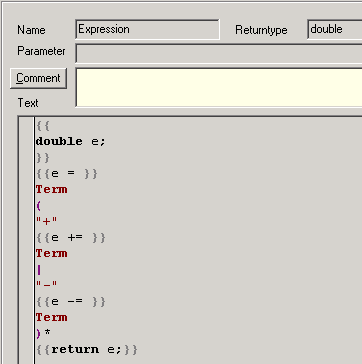Return values
 Examples > Calculator > Return values   The intermediate results of the calculator till now were delivered from reference variables from the invoked productions. Reference variables have the advantage, that they can be used in arbitrary number. In the calculator example, there always are only single intermediate results.   The calculator example using return values, is in the directory:   \TextTransformer\Examples\Calculator2   The start rule now is shortened to:   {{out << }} Expression {{out << endl;}}   The instruction for the output in the first line is incomplete according to the pure syntax of c++. Inside of the TextTransformer this notation is allowed, if immediately after the shift operator and the closing braces of the semantic action a call to a production follows. The called production must return a value - see below -, which can be written into the output.   The finishing action is:   out << endl;   endl is another notation for "\n", that means for a line break.     The Expression production in Calculator2 has no parameters but the return type: doubleA production (or token) with a return type must return a value of this type. This happens in the last line:   {{return e;}}   The double variable e is declared at the begin of the production. Its value gets the variable from the Term production in three lines, which again are shortened c++ instructions:   {{e = }} Term {{e += }} Term     {{e -= }} Term   The Term- and the Factor production are rewritten in the same manner as the Expression production, so that they use a return type instead of reference variables.   In the Number production the declaration of a temporary variable can be renounced, by the fact, that the return value of the stod function is passed on directly.   number {{ return stod(xState.str()); }}